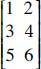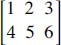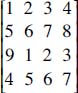# What are some examples of a matrix?

The dimensions of a matrix are the number of rows (horizontal numbers) and columns (vertical numbers); it is written as the rows first, columns second. The following are some simple examples of matrices—all differing in dimensions:

A 3 by 2 (3 × 2) matrix:A 2 by 3 (2 × 3) matrix:A 4 by 4 (4 × 4) matrix (when the row and column dimensions are the same, it is called a “square matrix”):Close

This is a web preview of the "The Handy Math Answer Book" app. Many features only work on your mobile device. If you like what you see, we hope you will consider buying. Get the App